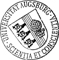## Water-wave scattering by vast fields of bodies

• A very efficient solution method to the determination of the linear water-wave scattering by a large number of bodies is presented. Several bodies are assembled in modules, which are grouped in periodic infinite line arrays. Then, using an iterative method, a finite number of these infinite arrays are stacked together. The method to calculate the scattering by the infinite line array of modules of bodies is algebraicly exact while a far-field (or wide-spacing) approximation is used in the calculation of the scattering of a finite stack of arrays. Bloch transmission through doubly periodic arrangements of bodies is discussed and so are averaging techniques to suppress phenomena introduced by the periodicity assumption on the line arrays for the case of more or less randomly distributed bodies. While the method is general and can be used in a variety of situations, the principle application of the method is to calculate the scattering by vast fields of ice floes which occur in theA very efficient solution method to the determination of the linear water-wave scattering by a large number of bodies is presented. Several bodies are assembled in modules, which are grouped in periodic infinite line arrays. Then, using an iterative method, a finite number of these infinite arrays are stacked together. The method to calculate the scattering by the infinite line array of modules of bodies is algebraicly exact while a far-field (or wide-spacing) approximation is used in the calculation of the scattering of a finite stack of arrays. Bloch transmission through doubly periodic arrangements of bodies is discussed and so are averaging techniques to suppress phenomena introduced by the periodicity assumption on the line arrays for the case of more or less randomly distributed bodies. While the method is general and can be used in a variety of situations, the principle application of the method is to calculate the scattering by vast fields of ice floes which occur in the Marginal Ice Zone. Preliminary numerical simulations for floating elastic plates, modelling ice floes, are presented and substantiate the applicability of the method.• Dokument_1.pdfAuthor: Malte A. PeterORCiDGND, Michael H. Meylan urn:nbn:de:bvb:384-opus4-10992 https://opus.bibliothek.uni-augsburg.de/opus4/1306 Preprints des Instituts für Mathematik der Universität Augsburg (2009-23) Preprint English Universität Augsburg Department of Mathematics, University of Auckland 2009/09/24 water waves; diffraction; multiple arrays; marginal ice zone; Bloch waves Meereswelle; Treibeis; Packeis; Hydroelastizität; Spektraltheorie; Streutheorie Mathematisch-Naturwissenschaftlich-Technische Fakultät Mathematisch-Naturwissenschaftlich-Technische Fakultät / Institut für Mathematik Mathematisch-Naturwissenschaftlich-Technische Fakultät / Institut für Mathematik / Lehr- und Forschungseinheit Angewandte Analysis 5 Naturwissenschaften und Mathematik / 51 Mathematik / 510 MathematikDeutsches Urheberrecht mit Print on Demand